# Basic theory

Consider a model for data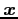assumed to be observed values of some random variable. This model defines a probability distribution forin terms of parameters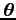, from a parameter space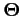, by a density function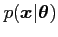. The value of this density datais often called the likelihood function as it describes the likelihood of this particular samplein terms of the parameters.

In a Bayesian model initial knowledge aboutis represented as a prior distribution having density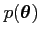. This may come from some `expert' assessment of the parameter value or from some previous measurement or experiment. Statistical inference foris obtained by using Bayes' Theorem to update knowledge aboutin the light of the sample data.

The inference about, given data, is provided by the posterior density given by Bayes' Theorem as: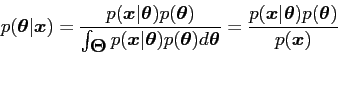(1)

whereis the entire parameter space.

It is often convenient and sufficient to express Bayes' theorem to proportionality1 as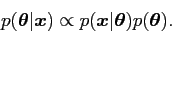(2)

Note that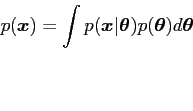(3)

is obtainable as the constant needed to make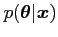a proper density, so that(4)

If the sample is large then the information contained in the prior is swamped by that in the data and the prior has little effect on the posterior density. If, on the other hand, the sample information is small the posterior will be dominated by the prior.

The Bayesian approach has several theoretical advantages over, for example, the more familiar frequentist methods. One such is that it does not violate the likelihood principle which implies that all the information to be learned aboutfrom the sample is captured in the likelihood (Lindley, 1965). Hence, two different samples having proportional likelihoods would have the same inference; this is true if Bayesian methods are used, see, for example, Savage (1962) and O'Hagan (1994). As a simple example of a statistic in common use that violates the likelihood principle consider the problem of estimating variance (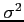)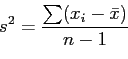(5)

where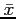sample mean and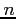sample size,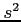is an unbiased estimator for. The denominator has been chosen to remove bias thus considering samples that have not been seen and, hence, information not in the observed data.

Subsections
danny 2009-07-23